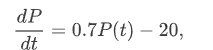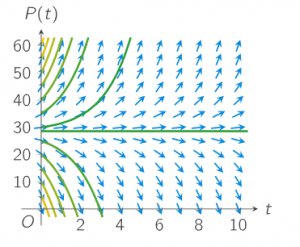# Calculation 1: Direction field & Equilibrium solution

Course subject(s) 1. Introducing Mathematical Modelling

In the previous section you have derived the differential equationstill with the initial condition P(0)=30.

Can you estimate the number of rainbowfish P(t) over time?

To answer this question, we will first construct a direction field, which is a special type of graph. In the next video, you will see how you can do this. In the video also some solutions are estimated. See for yourself!

## Direction fields

Sorry, there don't seem to be any downloads..

Subtitles (captions) in other languages than provided can be viewed at YouTube. Select your language in the CC-button of YouTube.

In the video you have seen how you can construct the direction field of our differential equation and how you can sketch solutions to the differential equation if you know the initial condition: